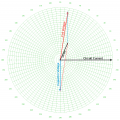# BASIC LC CIRCUIT QUESTION

#### themasoner

Joined Jan 29, 2016
1
Hey all,

Im getting back into the swing of things in electrical and im stuck on question could someone please give me a detail description on solving this question

A coil and a capacitor are connected in series across a 120V, 60HZ supply. The coil has an impedance of 232 ohms at a power factor angle of 81 degrees lagging. The capacitive reactance of the capacitor is 150 ohm. Calculate the voltage across the coil and the voltage across the capacitor

#### shteii01

Joined Feb 19, 2010
4,644
Convert inductor impedance into complex representation.

Convert capacitive reactance into capacitive impedance.

Now you have two "resistors". These two "resistors" are in series, therefore they form voltage divider, use voltage divider formula to find voltage across each.

#### WBahn

Joined Mar 31, 2012
28,490
Welcome to AAC.

In the future, please do not use all caps (I'm referring to your title, in this case) except for the occasional word. All caps is generally interpreted as shouting or, in the case of individual words, drawing emphasis (like bold font). The latter is fine, the former is generally considered rude. Live and learn.

As this is Homework Help and not Homework Done For You, please put forth your best attempt to tackle the problem. Don't worry if it isn't correct -- that gives us a starting point for the discussion.

#### RBR1317

Joined Nov 13, 2010
706
Tried to plot the description of the problem onto a phasor diagram. Since this is a series circuit, the same current flows in every component. Made the circuit current the phase reference at zero degrees. Don't know what the component voltages are, but the voltages are equal to I*Z so they are proportional to the impedance. Drew voltage lines that are proportional in length to the component impedance: coil voltage leading at 81 degrees, capacitor voltage lagging at -90 degrees. Although the component voltages are not known, we do know that their sum must equal the applied voltage. Anyway, that is how I understand the problem statement. Go forth and calculate.